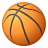# Convert Date to yyyymmdd string in field calculator with Python

5783
8
05-31-2019 05:16 AMOccasional Contributor

Hello,

I am stuck with syntax for what I think will be very easy task.  I have a feature class with a date field that I simply want to convert to a string field in yyyymmdd format, so that I can combine it with other fields to create a unique ID.

Id like to do this in a simple field calculator (in ArcMap) session with python

I tried using the strftime method with simple expression but that doesnt work   !mydatefield!.strftime('%Y%m%d').  I also tried a few other things but keep getting error

Any help would be greatly appreciated!

Thanks

Neal

Tags (1)
1 Solution

Accepted SolutionsbyMVP Esteemed Contributor

It always helps to provide the specific errors and tracebacks you are getting.  There are so many possible errors that just saying you are getting an error isn't helpful.

In ArcMap, cursors and the field calculator return date fields as text/unicode, so you have to be aware of that when trying to process the date information.  So you may need something like:

``(datetime.datetime.strptime( !DateField!,'%m/%d/%Y')).strftime('%Y%m%d')‍``
8 RepliesbyMVP Regular Contributor

This is kind of a long-winded answer, but it should give you the results you're looking for.  Since you did not specify, I assume your date field is in a format like this 5/31/2019.

``````date = str'5/31/2019'
ds = date.split('/')
formatted = ds + ds + ds

>>> formatted
'2019531'‍‍‍‍‍‍‍‍‍‍‍‍``````by
Occasional Contributor III

If you are starting with an actual datetime field [dt]:

``````import datetime
dt = datetime.datetime(2019, 5, 31, 0, 0)``````

`dt.strftime('``%Y%m%d``')`

or

``'{:%Y%m%d}'.format(dt)``

For completeness' sake: you can also directly access the attributes of the object, but then you only get the numbers:

``'%Y%m%d``' % (dt.month, dt.day, dt.year)``

``````For reference, here are the codes used in format string:

%a Weekday as locale’s abbreviated name.
%A Weekday as locale’s full name.
%w Weekday as a decimal number, where 0 is Sunday and 6 is Saturday.
%d Day of the month as a zero-padded decimal number.
%b Month as locale’s abbreviated name.
%B Month as locale’s full name.
%m Month as a zero-padded decimal number. 01, ..., 12
%y Year without century as a zero-padded decimal number. 00, ..., 99
%Y Year with century as a decimal number. 1970, 1988, 2001, 2013
%H Hour (24-hour clock) as a zero-padded decimal number. 00, ..., 23
%I Hour (12-hour clock) as a zero-padded decimal number. 01, ..., 12
%p Locale’s equivalent of either AM or PM.
%M Minute as a zero-padded decimal number. 00, ..., 59
%S Second as a zero-padded decimal number. 00, ..., 59
%f Microsecond as a decimal number, zero-padded on the left. 000000, ..., 999999
%z UTC offset in the form +HHMM or -HHMM (empty if naive), +0000, -0400, +1030
%Z Time zone name (empty if naive), UTC, EST, CST
%j Day of the year as a zero-padded decimal number. 001, ..., 366
%U Week number of the year (Sunday is the first) as a zero padded decimal number.
%W Week number of the year (Monday is first) as a decimal number.
%c Locale’s appropriate date and time representation.
%x Locale’s appropriate date representation.
%X Locale’s appropriate time representation.
%% A literal '%' character.
‍‍‍‍‍‍‍‍‍‍‍‍‍‍‍‍‍‍‍‍‍‍‍‍‍‍‍‍‍‍‍‍‍‍‍‍‍‍‍‍‍‍‍‍‍‍‍‍‍‍‍‍‍``````byMVP Esteemed Contributor

It always helps to provide the specific errors and tracebacks you are getting.  There are so many possible errors that just saying you are getting an error isn't helpful.

In ArcMap, cursors and the field calculator return date fields as text/unicode, so you have to be aware of that when trying to process the date information.  So you may need something like:

``(datetime.datetime.strptime( !DateField!,'%m/%d/%Y')).strftime('%Y%m%d')‍``Occasional Contributor

Hi Joshua - this helps thanks!

I wasnt putting the datetime.datetwice

INew Contributor II

I made this function for adding a string date field. Hope it helps someone:

``````def addStringDate(gdbname,tablename,datefieldname):
arcpy.AddField_management(in_table=gdbname+"/"+tablename, field_name=datefieldname+"_STR", field_type="TEXT", field_precision="", field_scale="", field_length="10", field_alias="", field_is_nullable="NULLABLE", field_is_required="NON_REQUIRED", field_domain="")
arcpy.CalculateField_management(in_table=gdbname+"/"+tablename, field=datefieldname+"_STR", expression="arcpy.time.ParseDateTimeString( !"+datefieldname+"!).strftime('%Y%m%d')", expression_type="PYTHON", code_block="")‍‍‍``````New Contributor III

With Field Calculator (python parser) you can convert a string field from a format using YYYYMMDD (example 20190522) to a date field (5/22/2019) using this expression:

(datetime.datetime.strptime( !OwnershipDate!,'%Y%m%d')).strftime('%m/%d/%Y')

My field named OwnershipDate is formatted as a string with values YYYYMMDD, in this example 20190522.

My reply is a bit redundant but after reading though many forum posts, everyone had slightly different requirements so the other examples threw me off.  This is for people who want to do what I specifically needed:   To calculate a date field from a string field formatted YYYYMMDD. Hope this adds clarity and helps someone!byMVP Esteemed Contributor

Be aware that cursors and field calculator return actual datetime objects in ArcGIS Pro.New Contributor II

Appears to return strings in Pro python though.  Thanks very helpful posts!